O Level Exam

Relational Operator in Python

 Symbol Description Example 1 Example 2 < Less than >>>7<10True>>> 7<5False>>> 7<10<15True>>>7<10 and 10<15True >>>"Hello"< "Goodbye"False>>>'Goodbye'< 'Hello'True > Greater than >>>7>5True>>>10<10False >>>„Hello‟> „Goodbye‟True>>>'Goodbye'> 'Hello'False <= less than equal to >>> 2<=5True>>> 7<=4False >>>"Hello"<= "Goodbye"False>>>'Goodbye' <= 'Hello'True >= greater than equal to >>>10>=10True>>>10>=12False >>>"Hello">= "Goodbye"True>>>'Goodbye' >= 'Hello'False ! =, <> not equal to >>>10!=11True>>>10!=10False >>>‟Hello‟!= "HELLO"True>>> "Hello‟ != "Hello‟False == equal to >>>10==10True>>>10==11False >>>"Hello" == "Hello"True>>>"Hello" == "Good Bye"False

Example: To test Relational Operators:

#Demo Program to test Relational Operators
a=int (input("Enter a Value for A:"))
b=int (input("Enter a Value for B:"))
print ("A = ",a," and B = ",b)
print ("The a==b = ",a==b)
print ("The a > b = ",a>b)
print ("The a < b = ",a<b)
print ("The a >= b = ",a>=b)
print ("The a <= b = ",a<=0)
print ("The a != b = ",a!=b)
#Program End

Output:

Enter a Value for A:35

Enter a Value for B:56

A = 35 and B = 56

The a==b = False

The a > b = False

The a < b = True

The a >= b = False

The a <= b = False

The a != b = True

Qus. What are operators? What is their function? Give examples of some unary and binary operators.

Ans: “Operators are those symbols used with operands, which tells compiler which operation is to be done on operands.‖ in other words – ―operators are tokens that trigger some computation/action when applied to variables and other objects in an expression.‖

Operators are of following types:

•  Unary operators like (+) Unary Plus, (-) Unary Minus, not etc.
•  Binary Operators like (+) addition, (*) multiplication, and etc.

Qus. What is block/code block/suit in Python?

Ans: Sometimes a group of statements is part of another statement of function. Such a group of one or more statements is called block or code-block or suit in python. e.g.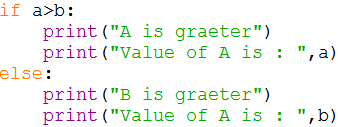Qus. What is the role of indentation in Python?

Ans: Indentation plays a very important role in Python. Python uses indentation to create blocks of code. Statements at same indentation level are part of same block/suit. You cannot unnecessarily indent a statement; python will raise an error for that.

Qus. How many types of strings are supported by Python?

Ans: Python supports two types of strings:

(i) Single-line string That terminates in single line.

(ii) Multi-line String That stores multiple lines of text.

Qus. What will be the sizes of following constants?

(a) "\a‟ (b) “\a” (c) “Kumar\‟s” (d) "\‟ (e) “it‟s”

Ans: (a) 50 (b) 50 (c) 56 (d) 50 (e) 53

This screenshot is the output of the above question.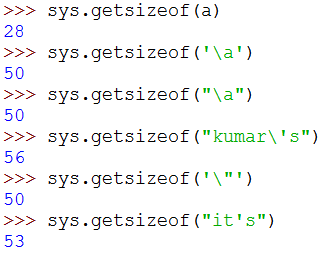Qus. How can you create multi-line strings in Python?

Ans: We can create multi-line string by putting a backslash (\) at the end of line which allows you to continue typing in next line in same string.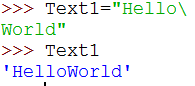Qus. Which of the following are syntactically correct strings? State reason.

(a) ”Python is nice Language”

(b) „He called me “Friend!” when he came‟

(c) “Very Good‟

(d) „This is a good book‟

(e) “Namaste

(f) “I liked „Harry Potter‟ very much”

Ans: (a) Correct (b) Correct (c) Incorrect (d) Correct (e) Incorrect (f) Correct

Qus. What is the error in following Python program with one statement?

print(“My name is : “, name)

suggest a solution

Ans: Error is : ―name 'name' is not defined‖. And the solution is to declare the variable-name before this statement.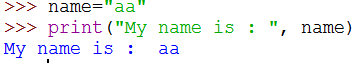Qus. Predict the output of the following: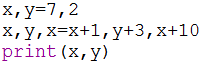Ans: Output: 17 5

Qus. What are augmented assignment operators? How are they useful?

Ans: An augmented assignment is generally used to replace a statement where an operator takes a variable as one of its arguments and then assigns the result back to the same variable. A simple example is x += 1 which is expanded to x = x + (1). Similar constructions are often available for various binary operators. They are helpful in making the source code small.

Qus. What are loops in Python? How many types of loop are there in Python?

Ans: Loops are iteration constructs in Python. Iteration means repetition of a set of statements depending upon a condition test. Loops has three basic elements within it to repeat the statements –

• Initialization (Start)
• Check Condition (Stop)
• Updation (Step)

Python provide two types of loop

(i) Conditional Loop while( (Condition based loop)

(ii) Counting loop for (loop for a given number of times).

Qus. What is the syntax of if-elif statement in Python?

Ans: The syntax of if-elif statement in python is as follows:

If condition1:

#code-block of statements when condition1 is true

elif condion2:

#code-block of statements when condition2 is true

elif condition3:

#code-block of statements when condition3 is true
.

.

.

else:

#code-block of statements when all above conditions are false.

Qus What are jump statements in Python? Name jump statements with example.

Ans: Python offers two jump statements to be used with in loops to jump out of loop-iterations.

These are break and continue statements.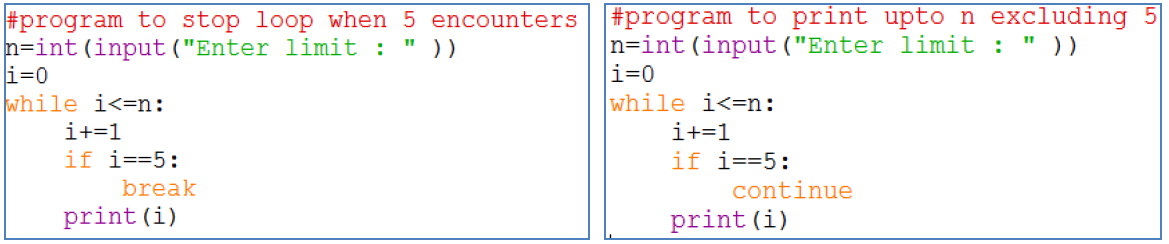Programs

Write a Program to obtain temperature in Celsius and convert it into Fahrenheit using formula –

C X 9/5 + 32 = F

WAP to read todays date (only date Part) from user. Then display how many days are left in the current month.

import datetime
td=0
day=int(input("Enter current date(only date part)"))
now=datetime.datetime.now()
if now.month==2:
td=28
elif now.month in(1,3,4,7,8,10,12):
td=31
else:
td=30
print("Total remaining days in the current month are : ",td-day)

WAP to print the area of circle when radius of the circle is given by user.

WAP to print the volume of a cylinder when radius and height of the cylinder is given by user.

area =πr2h

WAP that asks your height in centimeters and converts it into foot and inches.

WAP to accept 3 side of triangle and find area of a triangle.

WAP to input principle amount,rate time and calculate simple interest.

WAP to read a number in n and prints n^2, n^3, n^4

WAP to calculate compound simple interest after taking the principle, rate and time.

WAP to take two numbers and check that the first number is fully divisible by second number or not.

WAP to take value of x,y,z from the user and calculate the equation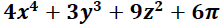WAP to take the temperatures of all 7 days of the week and displays the average temperature of that week.

WAP to check the given year is leap year or not.

WAP that searches for prime numbers from 15 through 25.

WAP to test if given number is prime or not.

WAP to compute the result when two numbers and one operator is given by user.

WAP to calculate the roots of a given quadratic equation.

WAP to input a digit and print it in words.

WAP to check whether square root of a given number is prime or not.

WAP to print first n odd numbers in descending order.

WAP to find the average of the list of the numbers entered through keyboard.

WAP to find the largest number from the list of the numbers entered through keyboard.

WAP to find the sum of n natural numbers.

WAP to find the sum of first n even numbers.

WAP to find the sum of first n odd numbers.

WAP a program to generate a list of elements of Fibonacci Series.

Program to find the simple interest based upon number of years. If number of years is more than 12 rate of interest is 10 otherwise 15.

Write a program to input any choice and to implement the following.

Choice Find

1. Area of square

2. Area of rectangle

3. Area of triangle

Write a program to input any number and to print all natural numbers up to given number.

Write a program to input any number and to find sum of all natural numbers up to given number.

Write a program to input any number and to find reverse of that number.

Write a program to input any string and count number of uppercase and lowercase letters.

Python program to obtain three numbers and print their sum.

Python program to obtain length and breath of a rectangle and calculate its area

Python program to calculate (BMI) body mass index of a person

Body Mass Index is a simple calculation using a person's height and weight. The formula is BMI=kg/m^2 where kg is a person's weight in kilogram and m^2 is the height in meter squared.

Write a program to input a number and print its cube.

Write a program to input two number and swap them.

Solution 1: Using Multiple Assignment

n1=int(input("Enter number 1 : "))
n2=int(input("Enter number 2 : "))
print("Original Number : ",n1,n2)
n1,n2=n2,n1
print("After Swapping : ",n1,n2)

Solution 2: Swapping by using 3rd variable

n1=int(input("Enter number 1 : "))
n2=int(input("Enter number 2 : "))
print("Original Number : ",n1,n2)
n3=n1
n1=n2
n2=n3
print("After Swapping : ",n1,n2)

Solution 3: Swapping by using Arithmetic Addition and Subtraction

n1=int(input("Enter number 1 : "))
n2=int(input("Enter number 2 : "))
print("Original Number : ",n1,n2)
n1=n1+n2
n2=n1-n2
n1=n1-n2
print("After Swapping : ",n1,n2)

Solution 4: Swapping by using Arithmetic Multiplication and Division

n1=int(input("Enter number 1 : "))
n2=int(input("Enter number 2 : "))
print("Original Number : ",n1,n2)
n1=n1*n2
n2=n1/n2
n1=n1/n2
print("After Swapping : ",n1,n2)

Write a program to input three numbers and swap them as this : 1st number becomes the 2nd number, 2nd number becomes the 3rd number and 3rd number becomes the first number.

Write a program to read three numbers in three variables and swap first two variables with the sums of first and second ,second and third number respectively.

Write a program to input a single digit(n) and print a 3 digit number created as <n(n+1)(n+2)> e.g., if you input 7, then it should print 789. Assume that the input digit is in range 1-7.

Write a program to accept a integer number from user and find its sum of digit.

Sample 1:

Input : 123

Output : Sum of Digit : 6

Sample 2:

Input : 1234

Output : Sum of Digit : 10

Write a python program to accept a integer number and count the number of digits in number.

Sample:

Input: 2223

Output: Number of Digits: 4

Write a Python program to accept a integer number form user and find the factorial value of input number.

The factorial of a number is the product of all the integers from 1 to that number. For example, the factorial of 6 is 1*2*3*4*5*6 = 720 . Factorial is not defined for negative numbers, and the factorial of zero is one, 0! = 1 .

Sample:

Input : 5

Output : 120

Write a Python program to input any number and print all factors of input number.

A factor is a number that divides into another number exactly and without leaving a remainder.

Sample:

Input : 12

Output : Factor of 12 are : 1,2,3,4,6 ,12

Write a Python program to accept a integer number from user and check number is composite Number or not.

Composite number is a whole numbers that have on 1 or more than 1 factors excluding 1 and itself.

Write a python program to accept a integer number form user and find the largest digit of number.

Sample:

Input : 12341

Output : Largest Digit is : 4

Write a python program to accept a integer number form user and find the smallest digit of number.

Sample:

Input : 12341

Output : Smallest Digit is : 1

write a program to print the table of a given number. The number has to be entered by the user.

Write a program to Calculate the Compound Interest

write a program to find sale price of an item with given price and discount (%)

write a program to calculate the sum of odd numbers divisible by 5 from the range 1..100

Write a Python program to accept 3 sides of triangle and find the area of triangle.

s = (a+b+c)/2

area = √(s(s-a)*(s-b)*(s-c))

Write a python program which accept two times as input and displays the total time after adding both the entered times.

Sample Input :

Enter Time 1 :

Hour : 3

Minute : 40

Enter Time 2 :

Hour : 2

Minute : 35

Output:

Time 1 : 3  Hour & 40  Minute

Time 2 : 2  Hour & 35  Minute

Total Time  : 6  Hour & 15  Minute

Write a python program which accept two times (HH:MM:SS) as input and displays the total time after adding both the entered times.

Sample :

Input:

Enter Time 1 :

Hour : 2

Minute : 40

Second : 45

Enter Time 2 :

Hour : 1

Minute : 35

Second : 40

Output:

Time 1 : 2  Hour, 40  Minute and  45  Second

Time 2 : 1  Hour, 35  Minute and  40  Second

Total Time  : 4  Hour & 16  Minute and  25 Second

write a program to print one of the words negative, zero or positive, according to whether variable x is less than zero, zero, or greater than zero, respectively.

Write a program that returns True if the input number is an even number, False otherwise.

Write a python program to input cost price, selling price of product from user and check whether is profit or loss and also print the Profit/loss amount

Write a python program to input a number form user and check whether number is buzz number or not.

A number is said to be Buzz Number if it ends with 7 or is divisible by 7.

Write a program to input assets, liabilities and capital of a company and test if accounting equation holds true for the given value (i.e., balanced or not).

Hint : assets == liabilities + capital

Write a program to input total debt and total assets and calculate total-debt-to-total-assets ratio (TD/TA) as Total Debt/Total Assets. Then print if the major portion of the debt is funded by assets (when TD/TA > 1) or greater portion of assets is funded by equity (when TD/TA < 1).

Write a program to calculate Debt-to-equity (D/E) ratio as [ Total Liabilities  / Total shareholders' ] equity after inputting total liabilities and total shareholders' equity. And then print if an investor should invest in the company or not. A D/E ratio greater than 2.0 indicates a risky scenario for an investor.

Write a python program to accept an integer number to find out and print the difference between greatest and smallest digits presents in the number .

Write a python program to accept an integer number and print the frequency of each digit present in the number .

Sample:

Input : 3255435

Output:

Digit Frequency

2 1

3 2

4 1

5 3

Write a program to print Floyd's triangle as shown below:

1

2  3

4  5  6

7  8  9  10

11 12 13 14 15

Write a program to input any number and to check whether given number is Armstrong or not.

Example:

Armstrong 153,

1^3+5^3 +3^3 =(1+125+27)=153

Write a program to find all prime numbers up to given number

Write a program to convert decimal number to binary.

Write a program to convert binary to decimal.

Program to print quotient.

Write a program to obtain principal amount, rate of interest and time from user and compute simple interest.

Program to calculate area and perimeter of a parallelogram

Program demonstrating working with power operator

Program of division operator(/)

Program of modulus

Program convert dollars in Rupee

Program to convert kilometers to miles

Program to convert the distance (in feet) to inches, yards,  and miles

Program to calculate GST

Credit Card Program

Program to print absolute value of number provided by the user.

Program to check divisibility of a number

Program to print the largest number

Program to display menu to calculate are of square or rectangle

Write a python program to print larger number using swap

x=int(input("Enter the first Number : "))
y=int(input("Enter the second Number : "))
if x>y:
x,y=y,x
print("The Larger Number is : ",y)
print("The smaller Number is : ",x)

Write Python program to calculate Electricity Bill

Write Python program to input three number and print in ascending order

Write a Python program to  check character is alphabetic character or not

Write Python program to print letters of entered word

Write a Python program to print ASCII code for entered message

Write Python program to print first ten mersenne numbers. Numbers in the form 2n-1 are called Mersenne numbers.

For example, the first Mersenne number is 21-1=1, the second Mersenne number is 22-1=3 etc.

Write a program to obtain x, y, z from user and calculate expression : 4x4 + 3y3 + 9z + 6π

Write a program that reads a number of seconds and prints it in form : mins and seconds, e.g., 200 seconds are printed as 3 mins and 20 seconds.

[Hint. use // and % to get minutes and seconds]

Write a program to take year as input and check if it is a leap year or not.

Write a program to take two numbers and print if the first number is fully divisible by second number or not.

Write a program to take a 2-digit number and then print the reversed number. That is, if the input given is 25, the program should print 52.

Solution

Write a program to take a 3-digit number and then print the reversed number. That is, if you input 123, the program should print 321.

Write a program to take two inputs for day, month and then calculate which day of the year, the given date is. For simplicity, take 30 days for all months. For example, if you give input as: Day3, Month2 then it should print "Day of the year : 33".

Write a program that asks a user for a number of years, and then prints out the number of days, hours, minutes, and seconds in that number of years.

How many years? 10

10.0 years is:

3650.0 days

87600.0 hours

5256000.0 minutes

315360000.0 seconds

Write a program that inputs an age and print age after 10 years as shown below:

In ten years, you will be 27 years old!

Write a program that generates six random numbers in a sequence created with (start, stop, step). Then print the mean, median and mode of the generated numbers.

Write a program to generate 3 random integers between 100 and 999 which is divisible by 5.

Write a program to generate 6 digit random secure OTP between 100000 to 999999.

Write a program to generate 6 random numbers and then print their mean, median and mode

Write a program to find a side of a right angled triangle whose two sides and an angle is given.

Write a program to calculate the radius of a sphere whose area (4πr2) is given.

Write a program to calculate the area of an equilateral triangle. (area = (√3 / 4) * side * side).

Write a program to input the radius of a sphere and calculate its volume (V = 4/3πr3)

Write a program to calculate amount payable after simple interest.

Write a program to input length of three sides of a triangle. Then check if these sides will form a triangle or not.

(Rule is: a+b>c;b+c>a;c+a>b)

Write a program to input 3 sides of a triangle and print whether it is an equilateral, scalene or isosceles triangle.

Write a program to take N (N > 20) as an input from the user. Print numbers from 11 to N. When the number is a multiple of 3, print "Tipsy", when it is a multiple of 7, print "Topsy". When it is a multiple of both, print "TipsyTopsy".

Write a program to input N numbers and then print the second largest number.

Given a list of integers, write a program to find those which are palindromes. For example, the number 4321234 is a palindrome as it reads the same from left to right and from right to left.

Write a complete Python program to do the following :

(ii) determine the number of digits n in X.

(iii) form an integer Y that has the number of digits n at ten's place and the most significant digit of X at one's place.

(iv) Output Y.

(For example, if X is equal to 2134, then Y should be 42 as there are 4 digits and the most significant number is 2).

Write a Python program to print every integer between 1 and n divisible by m. Also report whether the number that is divisible by m is even or odd.

m = int(input("Enter m: "))
n = int(input("Enter n: "))
for i in range(1, n) :
if i % m == 0 :
print(i, "is divisible by", m)
if i % 2 == 0 :
print(i, "is even")
else :
print(i, "is odd")

Write a Python program to input 2 numbers and fin the LCM.

Write a Python program to input two number and find the G.C.D

Write a program to input any Character and find its ASCII Value.

Write a Python program which accept a integer number form user and check number is perfect or Not.

A perfect number is a positive integer that is equal to the sum of its positive divisors, excluding the number itself.

For instance, 6 has divisors 1, 2 and 3, and 1 + 2 + 3 = 6, so 6 is a perfect number.

Perfect Numbers between 1 to 10000 are:

6,28,496,8128

Solution:

num=int(input("Enter any Number : "))
sum=0
for a in range (1,num):
if num%a==0:
sum=sum+a
if sum==num:
print(num ," is Perfect Number")
else:
print(num ," is not a Perfect Number")

Write a Python Program which accept a number form user and check number is Special or Not.

Special number is a integer number which where addition of sum of  digit and product of digits are same to number.

Example:

29:

2 + 9 = 11

2 x 9 = 18

11 + 18 = 29

Solution:

num=int(input("Enter any Number : "))
sum=0
product=1
temp=num
while num>0:
digit=num%10
sum=sum+digit
product=product*digit
num=num//10
if (product+sum)==temp:
print("it is Special Number ")
else:
print("it is not a Special Number ")

Write a Python program which accept the value of n from user and print first n Perfect Numbers.

n=int(input("Enter the value of N : "))
count=0
a=1;
while count<=n:
sum=0
for b in range (1,(a//2)+1):
if a%b==0:
sum=sum+b
if sum==a:
print(a ,end=' ')
count+=1
a+=1

Write a Python program using if Elif else statement to find the number of days present in a month:

month=eval(input("Enter Month Sr. No (ex: for Jan enter - 1 ): "))
if month==1 or month==3 or month==5 or month==7 or month==8 or month==10 or month==10 :
print("Number of days in Month are 31")
elif month==4 or month==6 or month==9 or month==11:
print("Number of days in Month are 30")
else:
print("Number of days in Month are 28 or 29")